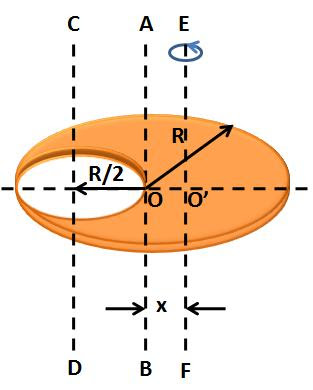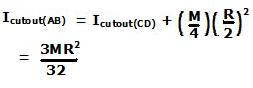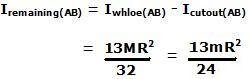## Tuesday, November 11, 2008

### Irodov Solution 1.241The mass of the remaining disc is m. Suppose that the mass of the original disc before it was cut out was M. The mass of portion that was cut out (of radius R/2) will be M/4.
In other words,

M - M/4 = m or,

M = (4/3)m.

The moment of inertia of a solid disc (before it was cut out) about axis AB that passes through its center and perpendicular to the plane of the disc is given by (see problem 1.240) .The moment of inertia of the portion of disc that was cut out about the axis CD (passing through its center) is given by,The moment of inertia of the cutout portion of the disc about an axis passing through AB can now be determined using the parallel axis theorem as,The moment of inertia of the remaining portion of the disc about AB can now be computed by simply subtracting the moment of inertia of the cut out portion disc from the that of the entire disc. In other words,Now the final twist to the tale. Irodov asks for moment of inertia about an axis that passes through the CG of the remaining portion of the disc and not the center of the disc. Since the portion of the disc was cut out, the CG of the remaining portion of the disc would have shifted to the right as indicated by O' in the figure. The mass of the portion of the disc that was cutout was M/4 and its CG is located at -R/2 (negative because its to the left) from O (the center of the original disc is considered as the origin). Suppose that the CG of the remaining part of the disc to O' located at x units from O. Then we have,Using the parallel axis theorem, now we can compute the moment of inertia of the remaining portion of the disc about EF (the axis passing through O') is given by,